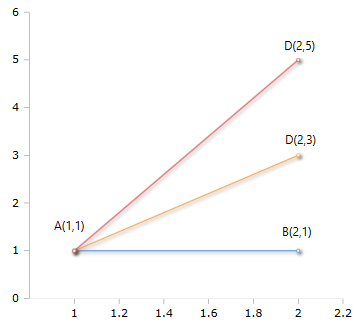Calculates the gradient (slope) of a straight line

### Description

The function $$Grad$$ calculates the gradient (slope) of a straight line between two points in the coordinate system. The end points of the lines are defined with their X / Y coordinates or two objects of the class Point.

### SyntaxBy using the $$Point$$ objects, the distance can also be calculated with arguments other than $$x / y$$. The class $$Point$$ contains its own methods for calculating the properties.
Suppose only the x-coordinates are known. $$y$$ is unknown, but the angles from the 0 point can be determined. In this case, the x-coordinate and the angle can be used as the point. An example of this can be found in the description of the Funbktion Distance.